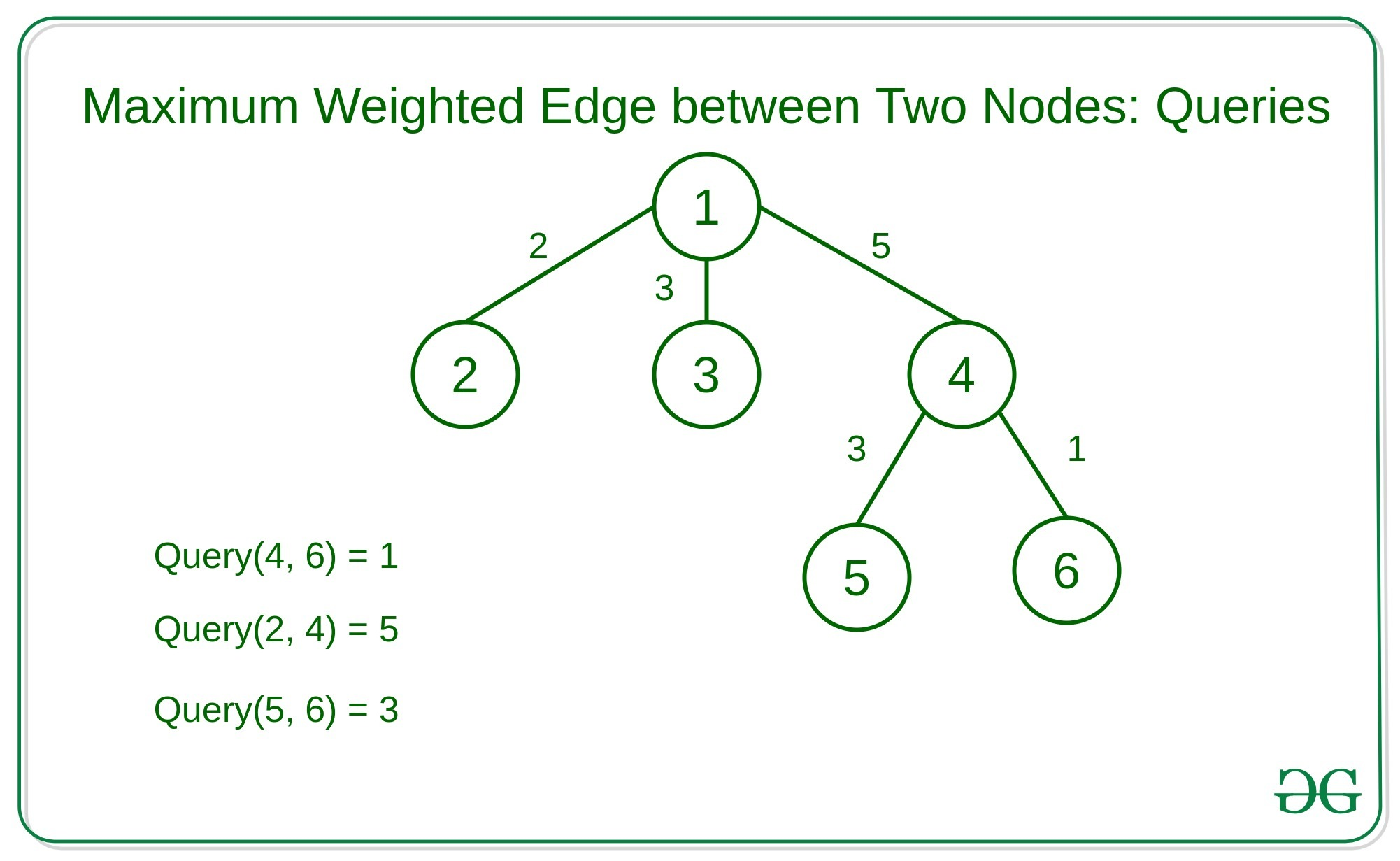Related Articles

# Maximum weighted edge in path between two nodes in an N-ary tree using binary lifting

• Difficulty Level : Hard
• Last Updated : 22 Jun, 2021

Given an N-ary tree with weighted edge and Q queries where each query contains two nodes of the tree. The task is to find the maximum weighted edge in the simple path between these two nodes.
Examples:Naive Approach: A simple solution is to traverse the whole tree for each query and find the path between the two nodes.
Efficient Approach: The idea is to use binary lifting to pre-compute the maximum weighted edge from every node to every other node at distance of some. We will store the maximum weighted edge tilllevel.andwhere

• j is the node and
• i is the distance of• dp[i][j] stores the parent of j at• distance if present, else it will store 0
• mx[i][j] stores the maximum edge from node j to the parent of this node at• distance.

We’ll do a depth-first search to find all the parents atdistance and their weight and then precompute parents and maximum edges at everydistance.
Below is the implementation of the above approach:

## C++

 `// C++ implementation to find the``// maximum weighted edge in the simple``// path between two nodes in N-ary Tree` `#include ` `using` `namespace` `std;` `const` `int` `N = 100005;` `// Depths of Nodes``vector<``int``> level(N);``const` `int` `LG = 20;` `// Parent at every 2^i level``vector > dp(LG, vector<``int``>(N));` `// Maximum node at every 2^i level``vector > mx(LG, vector<``int``>(N));` `// Graph that stores destinations``// and its weight``vector > > v(N);``int` `n;` `// Function to traverse the nodes``// using the Depth-First Search Traversal``void` `dfs_lca(``int` `a, ``int` `par, ``int` `lev)``{``    ``dp[a] = par;``    ``level[a] = lev;``    ``for` `(``auto` `i : v[a]) {` `        ``// Condition to check if its``        ``// equal to its parent then skip``        ``if` `(i.first == par)``            ``continue``;``        ``mx[i.first] = i.second;` `        ``// DFS Recursive Call``        ``dfs_lca(i.first, a, lev + 1);``    ``}``}` `// Function to find the ansector``void` `find_ancestor()``{` `    ``// Loop to set every 2^i distance``    ``for` `(``int` `i = 1; i < LG; i++) {``        ``// Loop to calculate for``        ``// each node in the N-ary tree``        ``for` `(``int` `j = 1; j <= n; j++) {``            ``dp[i][j]``                ``= dp[i - 1][dp[i - 1][j]];` `            ``// Storing maximum edge``            ``mx[i][j]``                ``= max(mx[i - 1][j],``                      ``mx[i - 1][dp[i - 1][j]]);``        ``}``    ``}``}` `int` `getMax(``int` `a, ``int` `b)``{``    ``// Swaping if node a is at more depth``    ``// than node b because we will``    ``// always take at more depth``    ``if` `(level[b] < level[a])``        ``swap(a, b);` `    ``int` `ans = 0;` `    ``// Difference between the depth of``    ``// the two given nodes``    ``int` `diff = level[b] - level[a];``    ``while` `(diff > 0) {``        ``int` `log` `= log2(diff);``        ``ans = max(ans, mx[``log``][b]);` `        ``// Changing Node B to its``        ``// parent at 2 ^ i distance``        ``b = dp[``log``][b];` `        ``// Subtracting distance by 2^i``        ``diff -= (1 << ``log``);``    ``}` `    ``// Take both a, b to its``    ``// lca and find maximum``    ``while` `(a != b) {``        ``int` `i = log2(level[a]);` `        ``// Loop to find the maximum 2^ith``        ``// parent the is different``        ``// for both a and b``        ``while` `(i > 0``               ``&& dp[i][a] == dp[i][b])``            ``i--;` `        ``// Updating ans``        ``ans = max(ans, mx[i][a]);``        ``ans = max(ans, mx[i][b]);` `        ``// Changing value to its parent``        ``a = dp[i][a];``        ``b = dp[i][b];``    ``}``    ``return` `ans;``}` `// Function to compute the Least``// common Ansector``void` `compute_lca()``{``    ``dfs_lca(1, 0, 0);``    ``find_ancestor();``}` `// Driver Code``int` `main()``{``    ``// Undirected tree``    ``n = 5;``    ``v.push_back(make_pair(2, 2));``    ``v.push_back(make_pair(1, 2));``    ``v.push_back(make_pair(3, 5));``    ``v.push_back(make_pair(1, 5));``    ``v.push_back(make_pair(4, 3));``    ``v.push_back(make_pair(3, 4));``    ``v.push_back(make_pair(5, 1));``    ``v.push_back(make_pair(3, 1));` `    ``// Computing LCA``    ``compute_lca();` `    ``int` `queries[]``        ``= { { 3, 5 },``            ``{ 2, 3 },``            ``{ 2, 4 } };``    ``int` `q = 3;` `    ``for` `(``int` `i = 0; i < q; i++) {``        ``int` `max_edge = getMax(queries[i],``                              ``queries[i]);``        ``cout << max_edge << endl;``    ``}``    ``return` `0;``}`

## Python3

 `# Python3 implementation to``# find the maximum weighted``# edge in the simple path``# between two nodes in N-ary Tree``import` `math``N ``=` `100005``;`` ` `# Depths of Nodes``level ``=` `[``0` `for` `i ``in` `range``(N)]``LG ``=` `20``;`` ` `# Parent at every 2^i level``dp ``=` `[[``0` `for` `j ``in` `range``(N)]``         ``for` `i ``in` `range``(LG)]`` ` `# Maximum node at every 2^i level``mx ``=` `[[``0` `for` `j ``in` `range``(N)]``         ``for` `i ``in` `range``(LG)]`` ` `# Graph that stores destinations``# and its weight``v ``=` `[[] ``for` `i ``in` `range``(N)]``n ``=` `0`` ` `# Function to traverse the``# nodes using the Depth-First``# Search Traversal``def` `dfs_lca(a, par, lev):` `    ``dp[``0``][a] ``=` `par;``    ``level[a] ``=` `lev;``    ` `    ``for` `i ``in` `v[a]:`` ` `        ``# Condition to check``        ``# if its equal to its``        ``# parent then skip``        ``if` `(i[``0``] ``=``=` `par):``            ``continue``;``        ``mx[``0``][i[``0``]] ``=` `i[``1``];`` ` `        ``# DFS Recursive Call``        ``dfs_lca(i[``0``], a, lev ``+` `1``);` `# Function to find the ansector``def` `find_ancestor():`` ` `    ``# Loop to set every 2^i distance``    ``for` `i ``in` `range``(``1``, ``16``):``    ` `        ``# Loop to calculate for``        ``# each node in the N-ary tree``        ``for` `j ``in` `range``(``1``, n ``+` `1``):``        ` `            ``dp[i][j] ``=` `dp[i ``-` `1``][dp[i ``-` `1``][j]];`` ` `            ``# Storing maximum edge``            ``mx[i][j] ``=` `max``(mx[i ``-` `1``][j],``                           ``mx[i ``-` `1``][dp[i ``-` `1``][j]]);` `def` `getMax(a, b):` `    ``# Swaping if node a is at more depth``    ``# than node b because we will``    ``# always take at more depth``    ``if` `(level[b] < level[a]):``        ``a, b ``=` `b, a`` ` `    ``ans ``=` `0``;`` ` `    ``# Difference between the``    ``# depth of the two given``    ``# nodes``    ``diff ``=` `level[b] ``-` `level[a];``    ` `    ``while` `(diff > ``0``):``        ``log ``=` `int``(math.log2(diff));``        ``ans ``=` `max``(ans, mx[log][b]);`` ` `        ``# Changing Node B to its``        ``# parent at 2 ^ i distance``        ``b ``=` `dp[log][b];`` ` `        ``# Subtracting distance by 2^i``        ``diff ``-``=` `(``1` `<< log);``     ` `    ``# Take both a, b to its``    ``# lca and find maximum``    ``while` `(a !``=` `b):``        ``i ``=` `int``(math.log2(level[a]));`` ` `        ``# Loop to find the maximum 2^ith``        ``# parent the is different``        ``# for both a and b``        ``while` `(i > ``0` `and``               ``dp[i][a] ``=``=` `dp[i][b]):``            ``i``-``=``1`` ` `        ``# Updating ans``        ``ans ``=` `max``(ans, mx[i][a]);``        ``ans ``=` `max``(ans, mx[i][b]);`` ` `        ``# Changing value to``        ``# its parent``        ``a ``=` `dp[i][a];``        ``b ``=` `dp[i][b];``    ` `    ``return` `ans;`` ` `# Function to compute the Least``# common Ansector``def` `compute_lca():``    ` `    ``dfs_lca(``1``, ``0``, ``0``);``    ``find_ancestor();` `# Driver code``if` `__name__``=``=``"__main__"``:``    ` `    ``# Undirected tree``    ``n ``=` `5``;``    ``v[``1``].append([``2``, ``2``]);``    ``v[``2``].append([``1``, ``2``]);``    ``v[``1``].append([``3``, ``5``]);``    ``v[``3``].append([``1``, ``5``]);``    ``v[``3``].append([``4``, ``3``]);``    ``v[``4``].append([``3``, ``4``]);``    ``v[``3``].append([``5``, ``1``]);``    ``v[``5``].append([``3``, ``1``]);`` ` `    ``# Computing LCA``    ``compute_lca();`` ` `    ``queries``=` `[[``3``, ``5``], [``2``, ``3``], [``2``,``4``]]``    ``q ``=` `3``;``    ` `    ``for` `i ``in` `range``(q):``        ``max_edge ``=` `getMax(queries[i][``0``],``                          ``queries[i][``1``]);``        ``print``(max_edge)``        ` `# This code is contributed by Rutvik_56`
Output:
```1
5
5```

Time Complexity:My Personal Notes arrow_drop_up